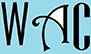## Algebra Question for Title 1 Schools: Simple Interest Question?

13) How many years will it take Henry to invest \$3,000 at 7% to make \$210 in simple interest?

I = (r x t x p) / 100

210 = (7 x t x 3000) / 100

you have to make “t” subject of formula by moving “everything else” to the other side. When you go to the other side, addition becomes subtraction, multiplication becomes division, and vice versa.

(210 x 100) / (7 x 3000) = t (100 was a division while 300 and 7 were multiplications at the other end)

21000 / 21000 = 1

t = 1 year